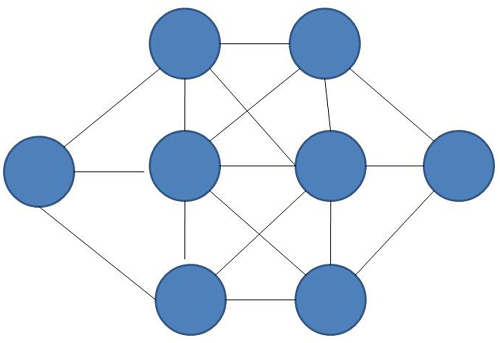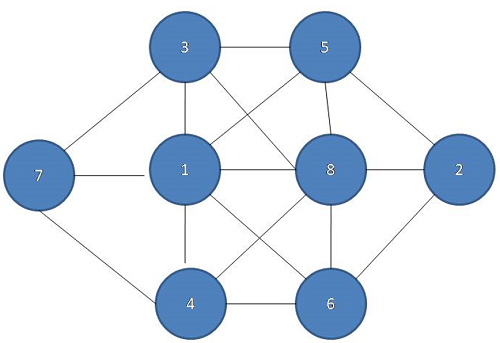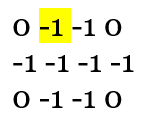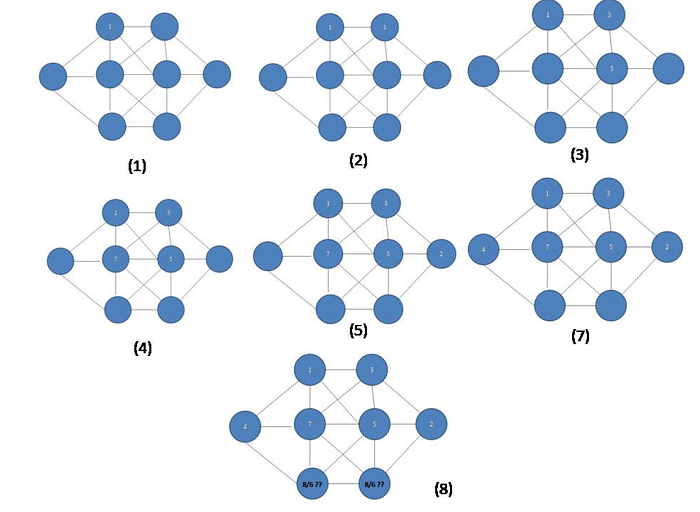# Fill 8 numbers in a matrix

Fill 8 numbers in a matrix: Here, we are going to learn to find the solution to to fill a matrix with 8 numbers using backtracking.
Submitted by Souvik Saha, on February 04, 2020

Description:

This is a standard interview problem to fill a matrix with 8 numbers using backtracking.

Problem statement:

A matrix is given to you and eight vacant spaces are there. You have to fill the places with the digits 1 to 8 in a way that there are not any adjacent places filled with its next digits.```    Input:
An matrix given to you

E.g.
0 -1 -1 0
-1 -1 -1 -1
0 -1 -1 0

Here -1 indicates the vacant places in the matrix.

Output:
Print the matrix with the numbers.
```

Example

```    If the matrix is this:
0 -1 -1 0
-1 -1 -1 -1
0 -1 -1 0

Output:
0 3 5 0
7 1 8 2
0 4 6 0
```Explanation with example

Placing the proper numbers in the right place is a try and error process and to solve it we use the backtracking process. To solve the matrix we have to follow the following steps.

2. Check how many numbers are left to fit for the place.
3. Start with any of the remaining numbers and check that its next numbers are not in its adjacent places.
4. Place the proper number in that vacant place and go for the adjacent vacant place.

If the matrix is this,And we start with the vacant place (1,2) and put the number 1 and if we will go (1,3), (2,3), (2,2), (2,4), (2,1) points followed by (1,2) and putting the numbers 3, 7, 2, 5. Then there are two vacant places but we will not fill those places with the numbers 8 or 6.So using the backtracking method, in the place of (2,3) will put the next number 6 and again applying the same method to fill all the vacant places with the numbers.

C++ implementation:

```#include <bits/stdc++.h>
using namespace std;

int x = { 0, 0, 1, -1, 1, -1, -1, 1 };
int y = { -1, 1, 0, 0, -1, -1, 1, 1 };

//check the validity of the point
bool is_valid(int row, int col, int a, int b)
{
return (a >= 0 && a < row && b >= 0 && b < col);
}

bool all_visited(int* mat, int row, int col)
{
int count = 0;

for (int i = 0; i < row; i++) {
for (int j = 0; j < col; j++) {
if (*(mat + i * col + j) == -1) {
return false;
}
}
}
return true;
}

bool print_matrix(int* mat, int row, int col)
{
for (int i = 0; i < row; i++) {
for (int j = 0; j < col; j++) {
cout << *(mat + i * col + j) << " ";
}
cout << endl;
}
return true;
}

bool is_safe(int* mat, int row, int col, int curr_row, int curr_col, int curr)
{
for (int j = 0; j < 8; j++) {
int x_axis = curr_row + x[j];
int y_axis = curr_col + y[j];

//checking for the next numbers
if (is_valid(row, col, x_axis, y_axis) && *(mat + x_axis * col + y_axis) != -1 && *(mat + x_axis * col + y_axis) != 0 && ((curr > 1 && (*(mat + x_axis * col + y_axis) == curr - 1 || *(mat + x_axis * col + y_axis) == curr + 1)) || (curr == 1 && *(mat + x_axis * col + y_axis) == curr + 1))) {
return false;
}
}
return true;
}

bool fill_matrix(int* mat, int row, int col, int curr_row, int curr_col, bool* visited, int prev)
{
int flag = 0;

for (int i = 1; i <= 8; i++) {
//if the point is not visited
if (!visited[i] && is_safe(mat, row, col, curr_row, curr_col, i)) {
visited[i] = true;
*(mat + curr_row * col + curr_col) = i;
if (all_visited(mat, row, col))
return true;
for (int j = 0; j < 8; j++) {
//go for the adjacents points
int x_axis = curr_row + x[j];
int y_axis = curr_col + y[j];
if (is_valid(row, col, x_axis, y_axis) && *(mat + x_axis * col + y_axis) == -1 && fill_matrix(mat, row, col, x_axis, y_axis, visited, i)) {
return true;
}
}
visited[i] = false;
*(mat + curr_row * col + curr_col) = -1;
}
}
return false;
}

int main()
{
int mat = { { 0, -1, -1, 0 },
{ -1, -1, -1, -1 },
{ 0, -1, -1, 0 } };

int flag = 0, prev = -1;
bool visited;

for (int i = 0; i < 9; i++) {
visited[i] = false;
}

for (int i = 0; i < 3; i++) {
for (int j = 0; j < 4; j++) {
if (mat[i][j] == -1) {
fill_matrix(&mat, 3, 4, i, j, visited, prev);
flag = 1;
break;
}
}
if (flag)
break;
}

//print the matrix
print_matrix(&mat, 3, 4);
return 0;
}
```

Output

```0 6 4 0
2 8 1 7
0 5 3 0
```

What's New (MCQs)

Top Interview Coding Problems/Challenges!

Languages: » C » C++ » C++ STL » Java » Data Structure » C#.Net » Android » Kotlin » SQL
Web Technologies: » PHP » Python » JavaScript » CSS » Ajax » Node.js » Web programming/HTML
Solved programs: » C » C++ » DS » Java » C#
Aptitude que. & ans.: » C » C++ » Java » DBMS
Interview que. & ans.: » C » Embedded C » Java » SEO » HR
CS Subjects: » CS Basics » O.S. » Networks » DBMS » Embedded Systems » Cloud Computing
» Machine learning » CS Organizations » Linux » DOS
More: » Articles » Puzzles » News/Updates

© https://www.includehelp.com some rights reserved.